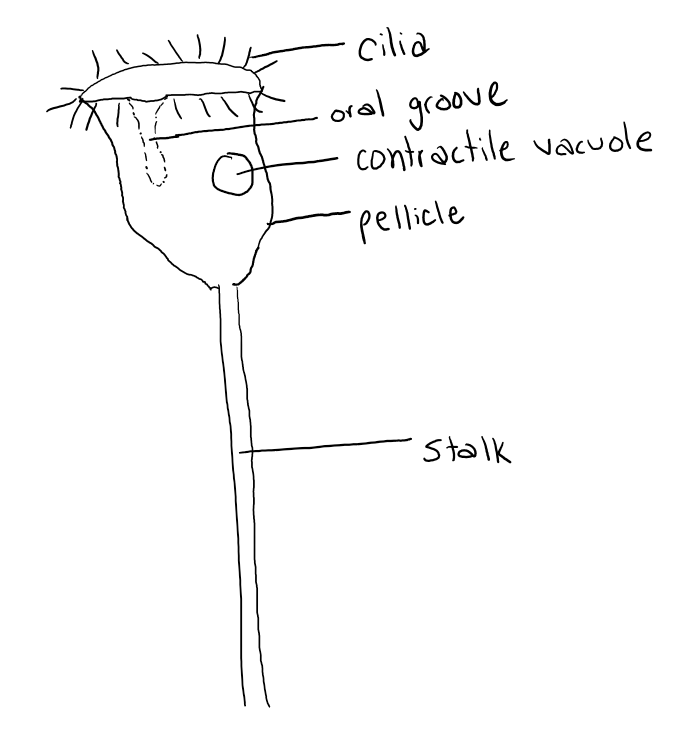## 17.5 Calculating Scale

Lastly, you can determine the drawing magnification using the following formula:

$m = \frac{d}{o}$

where

• $$m$$ = magnification (or scale) of drawing
• $$d$$ = size of drawing
• $$o$$ = size of object

Now that you know both the size of the drawing and the size of the object, you can plug that information into the above formula.

In the previous example (Under Estimating object size using field of view diameter), the protozoan drawing is 60 mm and the size of the protozoan was estimated to be 0.3 mm. Plugging these values into the formula, we get a magnification of 200x.

$m = \frac{60mm}{0.3mm} = 200$

Alternatively, you can write this as a ratio with drawing size: real life size. In this case the scale would be 200:1.

Place the scale or magnification on the bottom right of your drawing.

Units must match and then they cancel out, leaving the "x" to indicate magnification. You may also use the word "times" instead of "x".

Here is the final image.Whole (wet) mount of Vorticella campanula viewed at 400x total magnification using a brightfield compound microscope. Macronucleus not seen.
Image produced by Clerissa Copeland licensed under CC BY-NC-SA 4.0